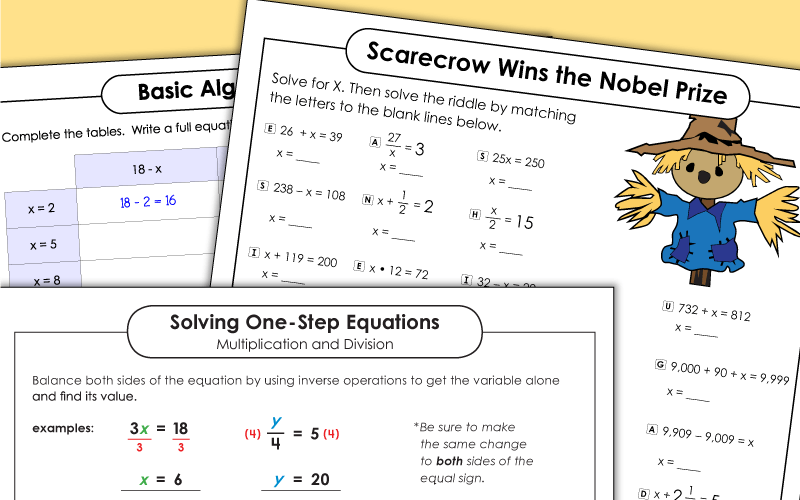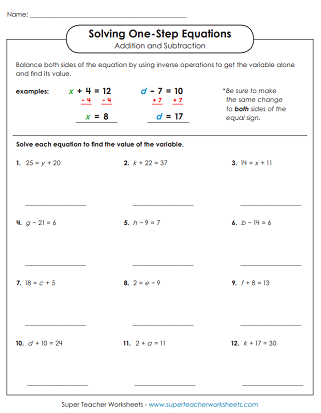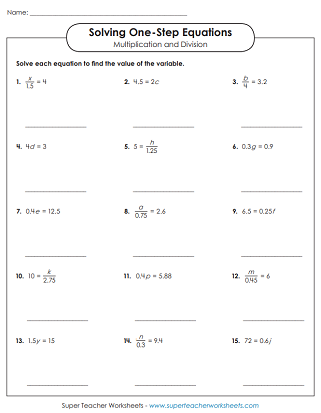# Algebraic Equations (Single Step)

On these printable activities, students will review solving algebraic equations with only one step. Common Core Standard 6.EE.B.7.## Solving for VariablesAddition & Subtraction Only

Solve each equation and find the value of the variable. This worksheet has a two model problems and 12 for students to solve. This basic level worksheet does not have decimals.
Find the value of x for each equation. These problems require only addition and subtraction.

## Solving for VariablesMultiplication & Division Only

Solve these basic multiplication and division equations. This version does not include decimals.
Use multiplication and division to find the value of each variable. This level includes decimal numbers.

Another worksheet in which students are required to find the value of the variables.
Solve the word problems. Write an equation and solve for the variable. This version uses only whole numbers.
Read the five scenarios and write a single-step equation for each.
Read the each scenario (word problem) and develop a one-step equation for each. This advanced-level worksheet features decimals.
Choose the best equation to solve each word problem.  Then, calculate the value of the variable.
Write an equation for each word problem. Then solve. This version includes decimals.
Complete the tables by substituting different numbers for variables.
Determine which algebraic equations are true, and which ones are not.
Why did the scarecrow win the Nobel Prize? To find out, find the value of x for each algebraic equation. Then match the letters with the numbers in the puzzle.
Students will use substitutions to find the value of a variable when 2 variables are given.
Students will use substitutions to solve for one of the two missing variables.

## Expressions & Equations Mixed

Mixed review page with different types of basic algebra problems
Learn to identify constants, variables, expressions, and equations.
More Pre-Algebra and Algebra

On this page, you'll link to a variety of pre-algebra and algebra topics, including writing expressions, evaluating expressions, one-step and two-step equations, inequalities, and more.

## Sample Worksheet ImagesMy Account
Site Information